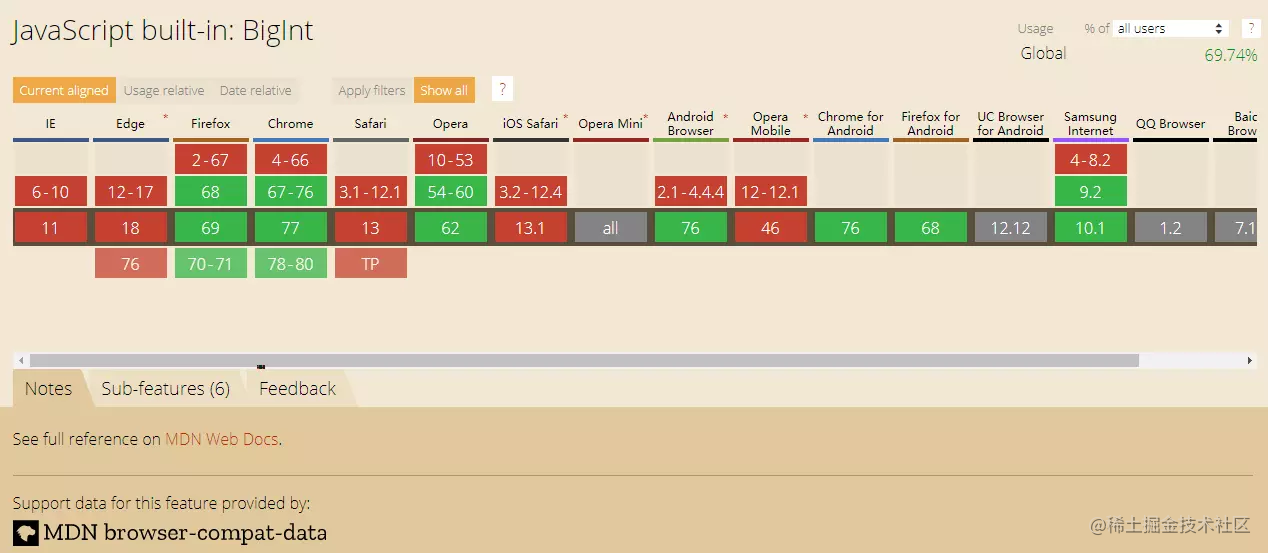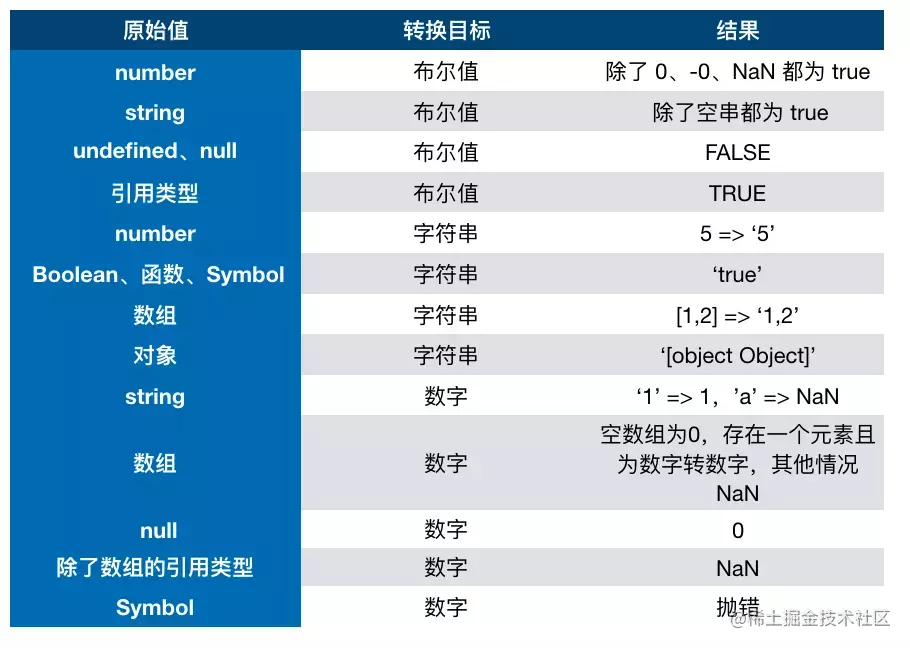# JS唬住面试官拿高薪 一 基础篇

## 第一章: JS数据类型之问——概念章

### JS原始数据类型有哪些？引用数据类型有哪些？

• 基本类型(值类型 原始值)，在JS中存在着 7 种，分别是：
• null
• undefined
• number
• string
• boolean
• bigint
• symbol
• 引用数据类型(对象类型), 包含:
• Object 普通对象
• Function 函数对象
• Array 数组对象
• Date 日期对象
• Math 数学函数
• RegExp 正则对象

### 说出下面运行的结果，解释原因

``````function test(person) {
person.age = 26
person = {
name: 'hzj',
age: 18
}
return person
}

const p1 = {
name: 'fyq',
age: 19
}
const p2 = test(p1)
console.log(p1) // -> ?
console.log(p2) // -> ?

/*
p1：{name: “fyq”, age: 26}
p2：{name: “hzj”, age: 18}
*/

### '1'.toString()为什么可以调用？

``````var s = new Object('1')
s.toString()
s = null

``````alert(6)
//等同于

### 0.1+0.2为什么不等于0.3？

0.1和0.2在转换成二进制后会无限循环，由于标准位数的限制后面多余的位数会被截掉，此时就已经出现了精度的损失，相加后因浮点数小数位的限制而截断的二进制数字在转换为十进制就会变成0.30000000000000004

### 如何理解BigInt?

##### 什么是BigInt?

BigInt是一种新的数据类型，用于当整数值大于Number数据类型支持的范围时。这种数据类型允许我们安全地对 大整数 执行算术操作，表示高分辨率的时间戳，使用大整数id，等等，而不需要使用库

##### 为什么需要BigInt?

``````console.log(999999999999999);  //=>10000000000000000

``````9007199254740992 === 9007199254740993;    // → true 居然是true!

##### 如何创建并使用BigInt？

``````console.log( 9007199254740995n );    // → 9007199254740995n
console.log( 9007199254740995 );     // → 9007199254740996

``````BigInt("9007199254740995");    // → 9007199254740995n

``````10n + 20n               // → 30n
10n - 20n               // → -10n
+10n                    // → TypeError: Cannot convert a BigInt value to a number
-10n                    // → -10n
10n * 20n               // → 200n
20n / 10n               // → 2n
23n % 10n               // → 3n
10n ** 3n               // → 1000n
const x = 10n
++x                     // → 11n
--x                     // → 9n
console.log(typeof x)   //"bigint"

##### 值得警惕的点
1. BigInt不支持一元加号运算符, 这可能是某些程序可能依赖于 + 始终生成 Number 的不变量，或者抛出异常。另外，更改 + 的行为也会破坏 asm.js代码。
2. 因为隐式类型转换可能丢失信息，所以不允许在bigint和 Number 之间进行混合操作。当混合使用大整数和浮点数时，结果值可能无法由BigInt或Number精确表示。
``````10 + 10n   // → TypeError

1. 不能将BigInt传递给Web api和内置的 JS 函数，这些函数需要一个 Number 类型的数字。尝试这样做会报TypeError错误
``````Math.max(2n, 4n, 6n)    // → TypeError

1. 当 Boolean 类型与 BigInt 类型相遇时，BigInt的处理方式与Number类似，换句话说，只要不是0n，BigInt就被视为truthy的值。
``````if (0n) { } //条件判断为false
if (3n) { } //条件为true

1. 元素都为BigInt的数组可以进行sort
2. BigInt可以正常地进行位运算，如| & >> << ^

### 浏览器兼容性

caniuse的结果:其实现在的兼容性并不怎么好，只有chrome67、firefox、Opera这些主流实现，要正式成为规范，其实还有很长的路要走。 我们期待BigInt的光明前途！

## 第二章: JS数据类型之问——检测章

### typeof 是否能正确判断类型？

``````typeof 1           // number
typeof '1'         // string
typeof undefined   // undefined
typeof true        // boolean
typeof Symbol()    // symbol
typeof null        // object

``````typeof []           // object
typeof {}           // object
typeof console.log  // function

``````const Person = function () { }
const p1 = new Person()
p1 instanceof Person    // true
var str1 = 'hello world'
str1 instanceof String  // false
var str2 = new String('hello world')
str2 instanceof String  // true

### instanceof能判断基本数据类型

``````class PrimitiveNumber {
static [Symbol.hasInstance](x) {
return typeof x === 'number'
}
}
console.log(111 instanceof PrimitiveNumber) // true

### 能不能手动实现一下instanceof的功能？

``````function instanceOf(left, right) {
let proto = left.__proto__
while (true) {
if (proto === null) return false
if (proto === right.prototype) {
return true
}
proto = proto.__proto__
}
}

``````function instanceOf(left, right) {
if (typeof left !== 'object' || left === null) return false   //基本数据类型直接返回false

let proto = Object.getPrototypeOf(left) //getProtypeOf是Object对象自带的一个方法，能够拿到参数的原型对象
while (true) {
if (proto == null) return false   //查找到尽头，还没找到
if (proto == right.prototype) return true //找到相同的原型对象
proto = Object.getPrototypeOf(proto)
}
}

console.log(instanceOf("111", String)) //false
console.log(instanceOf(new String("111"), String))//true

### Object.is和===的区别？

Object在严格等于的基础上修复了一些特殊情况下的失误，具体来说Onject.is()修复后就是+0 -0 不相等，NaN和NaN相等。 源码如下：

``````+0 === -0           //true
Object.is(+0,-0)    //false

NaN === NaN         //false
Object.is(NaN,NaN)  //true

``````function is(x, y) {
if (x === y) {
//运行到1/x === 1/y的时候x和y都为0，但是1/+0 = +Infinity， 1/-0 = -Infinity, 是不一样的
return x !== 0 || y !== 0 || 1 / x === 1 / y;
} else {
//NaN===NaN是false,这是不对的，我们在这里做一个拦截，x !== x，那么一定是 NaN, y 同理
//两个都是NaN的时候返回true
return x !== x && y !== y;
}

### JS用来判断类型最好的方法是什么？

``````Object.prototype.toString.call('')             // [object String]
Object.prototype.toString.call(1)              // [object Number]
Object.prototype.toString.call(true)           // [object Boolean]
Object.prototype.toString.call(Symbol())       // [object Symbol]
Object.prototype.toString.call(undefined)      // [object Undefined]
Object.prototype.toString.call(null)           // [object Null]
Object.prototype.toString.call(new Function()) // [object Function]
Object.prototype.toString.call(new Date())     // [object Date]
Object.prototype.toString.call([])             // [object Array]
Object.prototype.toString.call(new RegExp())   // [object RegExp]
Object.prototype.toString.call(new Error())    // [object Error]
Object.prototype.toString.call(window)         // [object global] window 是全局对象 global 的引用
Object.prototype.toString.call(document)       // [object HTMLDocument]

## 第三章: JS数据类型之问——转换章

### [] == ![] 结果是什么？为什么？

``````结果为true (0 == 0)

[]虽然作为一个引用类型转换为布尔值为 true

![] => false，进而在转换成数字变为 0

### JS中类型转换只有三种

• 转换成数字
• 转换成布尔值
• 转换成字符串### == 和 ===有什么区别？

=== 叫做严格相等，是指：左右两边不仅值要相等，类型也要相等

• 例如'1'===1的结果是false，因为一边是string，另一边是number

== 叫不严格相等，对于一般情况，只要值相等，就返回true，但==还涉及一些类型转换，它的转换规则如下：

• 两边的类型是否相同，相同的话就比较值的大小，例如1==2，返回false
• 判断的是否是null和undefined，是的话就返回true
• 判断的类型是否是String和Number，是的话，把String类型转换成Number，再进行比较
• 判断其中一方是否是Boolean，是的话就把Boolean转换成Number，再进行比较
• 如果其中一方为Object，且另一方为String、Number或者Symbol，会将Object转换成字符串，再进行比较
``````console.log({a: 1} == true);//false
console.log({a: 1} == "[object Object]");//true

### 对象转原始类型是根据什么流程运行的？

1. 如果Symbol.toPrimitive()方法，优先调用再返回
2. 调用valueOf()，如果转换为原始类型，则返回
3. 调用toString()，如果转换为原始类型，则返回
4. 如果都没有返回原始类型，会报错
``````var obj = {
value: 1,
[Symbol.toPrimitive]() {
return 2
},
valueOf() {
return 3
},
toString() {
return '4'
},
}
console.log(obj + 1) // 输出7

### 如何让if(a == 1 && a == 2)条件成立？

``````var a = {
value: 0,
valueOf: function() {
this.value++;
return this.value;
}
};
console.log(a == 1 && a == 2); //true